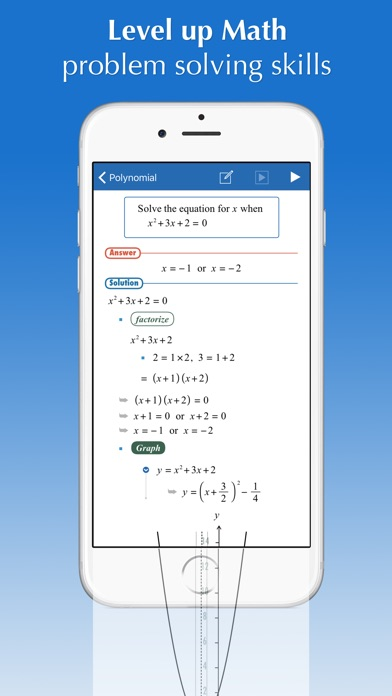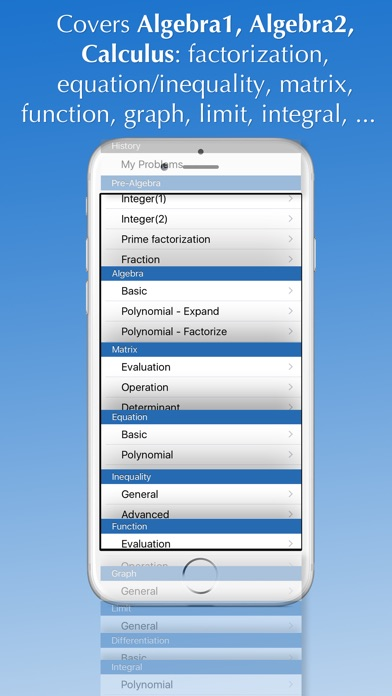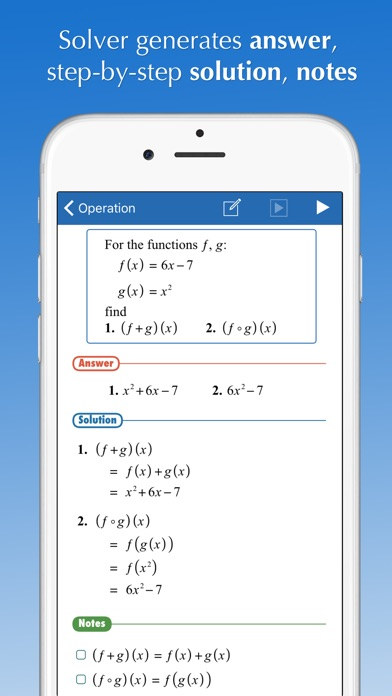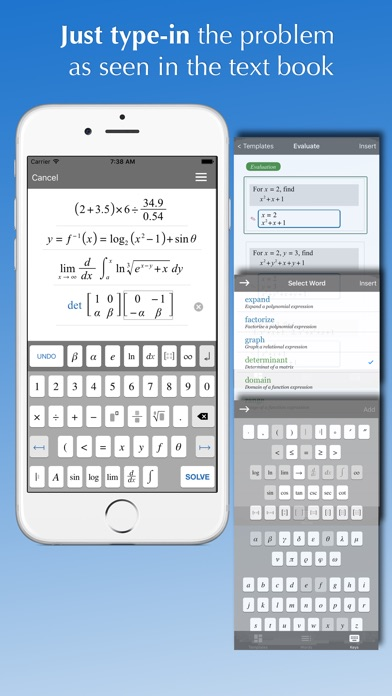# Quanrel

Navigation

## Related Articles

Filter by Category

Filter by Author

# FX Math Solver

quanrel
FX Math Solver

Will guide you how to solve your math homework and textbook problems, anytime, anywhere.

FX Math Solver is a comprehensive math software, based on an automatic mathematical problem solving engine,
and ideal for students preparing term math exams, ACT, SAT, and GRE:

– Over 1,500 sample math problems and fully animated solution steps
– Scientific calculator supported
– Graphing calculator supported
– Automatic problem solving and generation of fully animated step-by-step procedures for problems typed in by users
– User friendly math problem expression editor (WYSIWYG mode)

Math Solver covers problems at the level of Pre-Algebra, Algebra1, Algebra2, and Calculus courses:

– Number operations: add, subtract, multiply, divide
– Prime factorization
– Mixed number
– Complex number

– Basic expression simplification:
– polynomial – factorization, long-division
– rational
– radical
– exponential and log/ln
– sin, cos, tan, ..
– Matrix
– Equations, system of equations
– Inequalities

– Function

– Graphing

– Limit

– Differentiation

– IntegralFind out more

Show Comments (0)

### Related Articles

#### Instagram

Instagram is a simple way to capture and share the world’s moments. Follow your friends and family to see what they’re up to, and discover accounts from all over the world that...

Posted on by quanrel

#### Doodle Jump – Insanely Good!

Play this FREE version of the mega-hit game Doodle Jump and find out for yourself what millions of players around the world already know: DOODLE JUMP IS INSANELY ADDICTIVE!...

Posted on by quanrel